Next: 4.1.1 Scaled Energy Dispersion Up: 4. Quantum Confinement and Previous: 4. Quantum Confinement and

# 4.1 Quantization in UTB Films for Unprimed Subbands

In the following I will concentrate on the analytical analysis of the problem, allowing computationally cheap results, while offering an already detailed characterization of the subband structure in the Ultra Thin Body (UTB) silicon films behavior under uniaxially strain. However, the used assumption of a square well potential with infinite walls is only valid as long as the ground subband energy is much higher than the amplitude of the potential profile in the film. As soon as this assumption breaks down, a generalized numerical treatment of the k.p Schrödinger and Poisson equations is required.

The two-band k.p Hamiltonian of a  valley in the vicinity of the X-point of the Brillouin zone in Si must be in the form :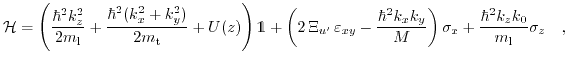(4.1)

where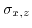are the Pauli matrices,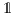is the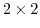unity matrix,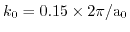is the position of the valley minimum relative to the X-point in unstrained Si,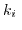with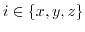is the wave vector,denotes the shear strain component in physics notations,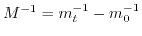, and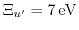is the shear strain deformation potential [161,170,179,180].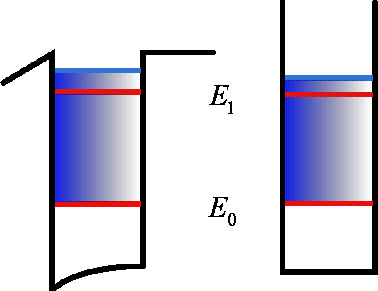For a square well potential the wave function is set to zero at the boundaries, which allows an analytical analysis of the subband structure.

In the two-band model the wave function is a spinor with two components. Therefore, we use the following ansatz,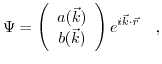(4.2)

whereand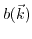are constants depending on the wave vector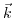. Substituting this ansatz into the equation system (4.1) delivers the following eigenvalue problem: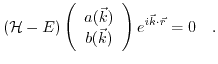(4.3)

Taking the determinant of (4.3) and setting it to zero results in the energy dispersion relation of the system.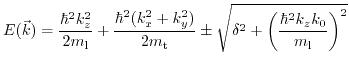(4.4)

with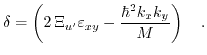(4.5)

For each energy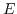there are four solutions for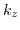. Fig. 4.2 shows that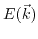is even with respect to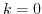. Therefore, there are always two independent values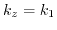and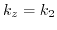for the wave vector, which are complemented to four values by alternating their signs. For energies in the gap the two values are imaginary. The wave function is then a superposition of the solutions with the four eigenvectors: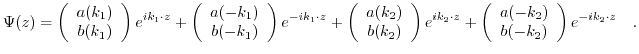(4.6)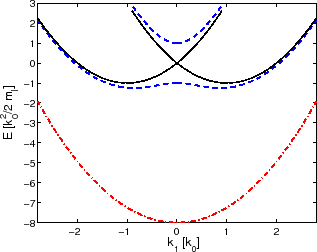We introduce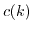as the ratio between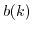and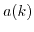.is an odd function with respect to: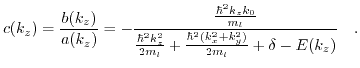(4.7)

Additionally, fulfilling the boundary conditionsdemands that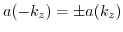is satisfied. Thus resulting in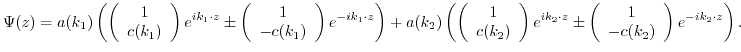(4.8)

After some simplifications the two pairs of independent equations(4.9)

and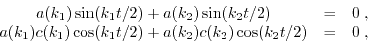(4.10)

are obtained.

Expressing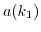with (4.9) and putting them into (4.10) leads to these two conditions: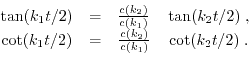(4.11)

After transforming the equations into dimensionless form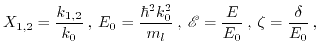(4.12)

and a few calculation steps found in the Appendix A., the equations can be written in the form: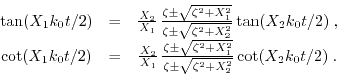(4.13)

For the numerical solution it is convenient to reformulate the equations as: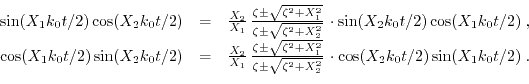(4.14)and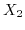coexist in the equations. Therefore, we need an extra relation to re-expressas a function ofor vice versa, the derivation of which can be found in the Appendix A.: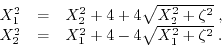(4.15)

Eliminating one of the two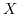's with (4.15) in (4.14) allows to calculateas a function of strain. Then one can calculate the energy as a function of strainby using (4.4).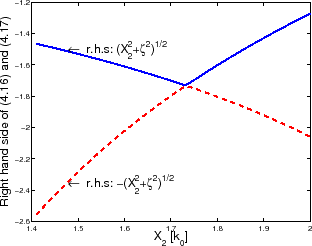Interestingly, (4.13) coincides with the dispersion relations obtained from an auxiliary tight-binding consideration . For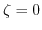(4.13) become equivalent. For higher strain values (4.14) must be solved numerically. The value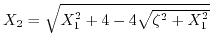becomes imaginary at high strain values. In this case the trigonometric functions in (4.13) or (4.14) are replaced by the hyperbolic ones. Special care must be taken to choose the correct branch of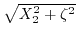in (4.13) or alternatively (4.14). The sign ofmust be alternated after it becomes zero, as it is displayed in Fig. 4.3.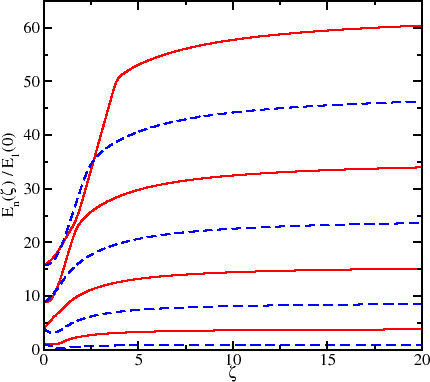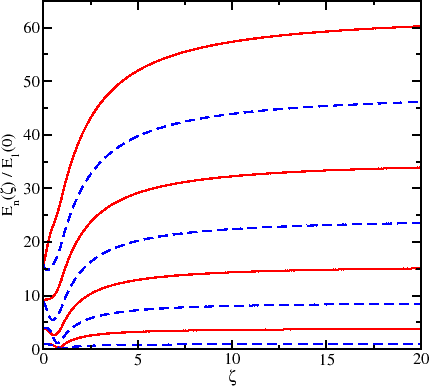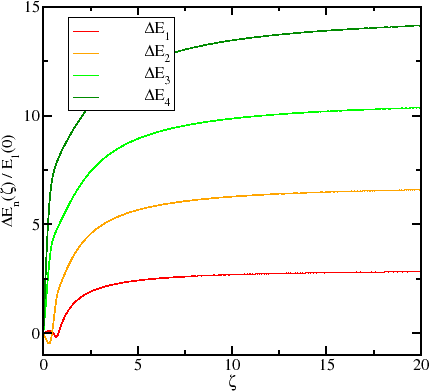Fig. 4.4 and Fig. 4.5 show the energies of the subbands as a function of shear strain for two different film thicknesses. Shear strain opens the gap between the two conduction bands at the X-point making the energy dispersion non-parabolic , which removes the subband degeneracy and introduces the valley splitting. Fig. 4.6 and Fig. 4.7 show the energy difference between two unprimed subbands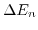as a function of strain for the same quantum number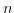.

SubsectionsNext: 4.1.1 Scaled Energy Dispersion Up: 4. Quantum Confinement and Previous: 4. Quantum Confinement and

T. Windbacher: Engineering Gate Stacks for Field-Effect Transistors